#Quality Digest

Featured Product
This Week in Quality Digest Live
Statistics Features
Donald J. Wheeler
Process-hyphen-control illustrated
Donald J. Wheeler
What does this ratio tell us?
Harish Jose
Any statistical statement we make should reflect our lack of knowledge
Donald J. Wheeler
How to avoid some pitfalls
Kari Miller
CAPA systems require continuous management, effectiveness checks, and support
Statistics News
How to use Minitab statistical functions to improve business processes
New capability delivers deeper productivity insights to help manufacturers meet labor challenges
Day and a half workshop to learn, retain, and transfer GD&T knowledge across an organization
Elsmar Cove is a leading forum for quality and standards compliance
InfinityQS’ quality solutions have helped cold food and beverage manufacturers around the world optimize quality and safety
User friendly graphical user interface makes the R-based statistical engine easily accessible to anyone
Collect measurements, visual defect information, simple Go/No-Go situations from any online device
Good quality is adding an average of 11 percent to organizations’ revenue growth
Ability to subscribe with single-user minimum, floating license, and no long-term commitmentBio

Statistics

## Some Outlier Tests: Part 2

### Tests with fixed overall alpha levels

Published: Monday, January 11, 2021 - 13:03

All articles in this series:

In part one we found the baseline portion of an XmR chart to be the best technique for identifying potential outliers among four tests with variable overall alpha levels. In this part we will look at tests which maintain a fixed overall alpha level regardless of how many values are being examined for outliers.

Before we get lost in the mechanics of detecting outliers it is important to think about the big picture. The objective of data analysis is not to compute the “right” numbers, but rather to gain the insight needed to understand what the data reveal about the underlying process. If the outliers are simply anomalies created by the measurement process, then they should be deleted before proceeding with the computations. But if the outliers are signals of actual changes in the underlying process represented by the data, then they are worth their weight in gold because unexpected changes in the underlying process suggest that some important variables have been overlooked. Here the deletion of the outliers will not result in insight. Instead, insight can only come from identifying why the unexpected changes happened. Nevertheless, whether we delete the outliers and proceed with our statistical computations, or stop to learn why the outliers happened, the first step is still the detection of the outliers.

### Tests with fixed overall alpha levels

The tests covered in part one are completely determined by the number of values in the data set being tested. No choices on the part of the user were required. The tests considered here will depend upon both the number of values in the data set and the user’s choice for the overall risk of a false alarm. So how do you make this choice?

Option One: If you think that one or more outliers are likely to be present in your data, then you will want to use an overall alpha of 10 percent. Such tests will detect more potential outliers than those with smaller alpha levels. About one time in 10 these tests will give you a single false alarm, and about nine times out of 10 they will have no false alarms. These tests will generally have a positive predictive value (PPV) value in the neighborhood of 88 percent. That is, the potential outliers identified will have about an 88-percent chance of being real outliers. So, when you are skeptical about the quality of the data, use an alpha level of 10 percent.

Option Two: If you do not know whether your data may or may not have any outliers, then use the traditional overall alpha level of 5 percent. You may find fewer potential outliers, but the larger outliers will still show up. Only about one test in 20 will have a single false alarm, and the general PPV values for potential outliers will be around 92 percent.

Option Three: If you are virtually certain that your data contain no outliers, then you may use an overall alpha level of 1 percent. Here you can almost completely avoid false alarms while still checking for the presence of large outliers. Typical PPV values here are around 97 percent. Use this option only when finding outliers is a low priority.

### The ANOX test for outliers

The analysis of individual values (ANOX) was developed by this author and James Beagle in 2017 as an extension of the XmR chart test for outliers1. Here the limits provide for a fixed risk of a false alarm. The ANOX test for outliers uses limits of:

Average  ±  ANOXalpha* Average Moving Range

Where the ANOX scaling factor depends upon both the number of values, n, and the user’s choice of alpha level. These values are tabled below. (More extensive tables are available in 1.)

The risk that the single most extreme value in a set of n data will fall outside these limits by chance is defined by the stated alpha level. For this reason, any and all points that fall outside the ANOX limits may be reasonably interpreted as outliers.

Figure 1 gives the ANOX scaling factors for an alpha level of 10 percent. This table should be used when you suspect that outliers may be present since it will identify more potential outliers than the other tables while holding the risk of a single false alarm to only 10 percent.Figure 1: 10-percent ANOX scaling factors

Figure 2 gives the ANOX scaling factors for an alpha level of 5 percent. This table holds the risk of a single false alarm to only 5 percent, and may be used when you suspect outliers to be less likely.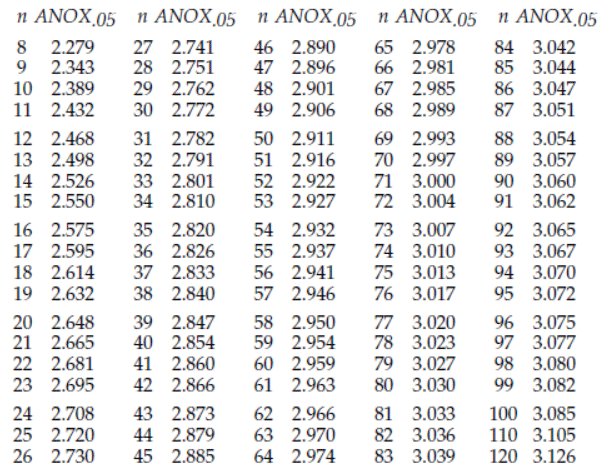Figure 2: 5-percent ANOX scaling factors

Figure 3 gives the ANOX scaling factors for an alpha level of 1 percent. This table holds the risk of a single false alarm to only 1 percent. It is biased against finding any outliers in favor of including all the data as good data. It should be used only when you are highly confident that there are no outliers in your data.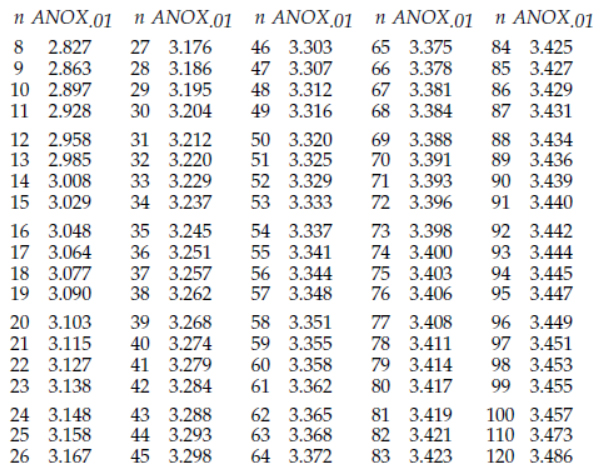Figure 3: 1-percent ANOX scaling factors

The PPV curves for the ANOX tests for outliers are shown in figure 4 along with the PPV curve for the XmR chart from part one.Figure 4: PPV curves for ANOX test for outliers

So, whenever you use a 10-percent ANOX to test for outliers, the points you identify as potential outliers have an 88-percent chance of being real outliers that do not belong with the rest of your data.

When you use a 5-percent ANOX test, you may not find as many potential outliers as with a 10-percent ANOX, but the potential outliers you do find will have a 92-percent chance of being real outliers.

When you use a 1-percent ANOX, you will find fewer potential outliers than with a 5-percent ANOX, but those you do identify will have a 97-percent chance of being real outliers.

In contrast to these ANOX tests with their fixed alpha levels, the XmR chart will behave something like a 1-percent ANOX test when n < 12, similar to a 5-percent ANOX test when 13 < n < 30, and something ike a 10-percent ANOX test when n ≥ 30. Thus, the 5-percent ANOX and 10-percent ANOX tests will be more sensitive to outliers than the XmR test when n is small.

Since, like the XmR chart, the ANOX test uses the average moving range it also cannot be used on data that have been arranged in ascending or descending order. While the time order for the data is the preferred ordering, any arbitrary ordering that is independent of the values for the data may be used with the ANOX test.

One other limitation exists for the ANOX test, and this is the limitation imposed by chunky data. As long as the average moving range is greater than 0.9 measurement increments the ANOX test will work as advertised. When the average moving range drops below 0.9 measurement increments the chunkiness of the data will begin to cause the limits to shrink due to roundoff effects. This shrinkage will increase the number of false alarms.

So ANOX combines the simplicity of the XmR chart with the advantage of being able to choose in advance a fixed overall alpha level for your test.

### Grubbs’ test for outliers

In 1950, Frank E. Grubbs published a test for identifying outliers2. His test is equivalent to the following: Given n data (n ≥ 4) compute the average and the global standard deviation statistic. Let G(n,α) be defined by:where the symbol t denotes the critical value from a Student’s t-distribution with [n–2] degrees of freedom which cuts off an upper tail area equal to [α/2n].

Grubbs’ test uses the interval:

Average ± G(n,α) * Standard Deviation Statistic

Any and all values outside this interval are designated as outliers. Figure 5 gives selected values of G(n,α).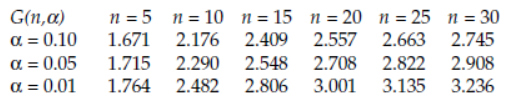Figure 5: Selected G(n,α) values for Grubbs’ test for outliers

Figure 6 lists the PPV values for the Grubbs’ cutoffs given in figure 5. Figure 7 shows the PPV curves for Grubbs’ test.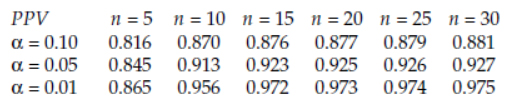Figure 6: PPV values for Grubbs’ test for outliers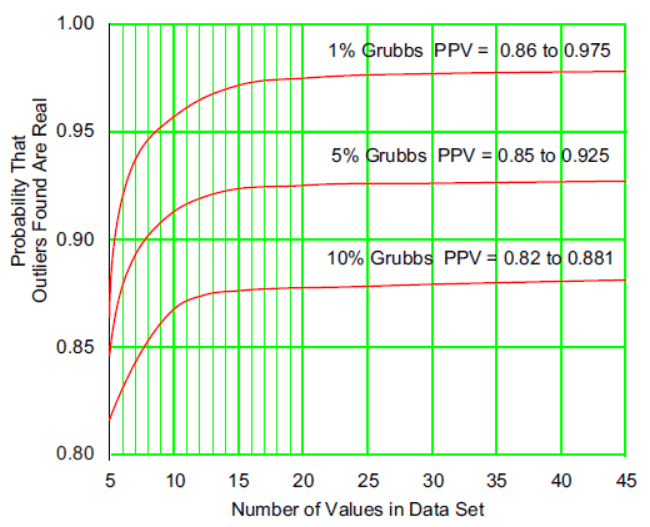Figure 7: PPV curves for Grubbs’ test for outliers

These PPV curves are very close to those found for the ANOX test, which suggests fairly equivalent performance. Thus, both the ANOX test and Grubbs’ test will allow you to test for outliers using a fixed overall alpha level that will result in a reasonable degree of belief that the outliers identified are indeed real.

### Limitations

Most tables of the Grubbs’ critical values include values for n = 3. These critical values are theoretical values derived under the assumption that the measurements are observations drawn from a continuum. Yet in practice, all data display some level of chunkiness, and this chunkiness places some limitations on Grubbs’ test.

We start by recalling from Shiffler3 that there is a maximum standardized value for a set of n data of: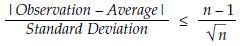This means that it will be impossible for any observation to ever fall outside the interval:For n = 3 this upper bound for a standardized value is 1.1547. Coincidentally, the 1 percent critical value for Grubbs’ test for n = 3 is G(3, 0.01) = 1.1547. Thus, with three observations, it is impossible to ever get a value that will exceed the 1 percent Grubbs’ cutoff. Hence, for n = 3 Grubbs’ test with alpha = 0.01 will never detect an outlier!

For alpha = 0.05 and n = 3 the Grubbs’ critical value is G(3,0.05) = 1.1543. In order to get one standardized value in between 1.1543 and 1.1547, a difference of 0.0004, the standard deviation will have to allow increments of 0.0002 in the standardized values. When we invert this number we discover that the standard deviation will have to exceed 5,000 measurement increments! Unless the standard deviation is greater than 5,000 measurement increments it will be impossible to compute a standardized value in between the critical value of 1.1543 and the upper bound of 1.1547. And if we cannot compute a value that falls in this interval, the test will never detect an outlier.

For alpha = 0.10 and n = 3 the Grubbs’ critical value is G(3, 0.10) = 1.1531. To allow a standardized value to fall halfway in between this critical value and the upper bound of 1.1547, the standard deviation will have to allow increments of 0.0008 in the standardized values. Inverting this we find that the standard deviation will have to exceed 1,250 measurement increments before we can begin to use Grubbs’ test for n = 3 and alpha = 0.10. Since it is rare to find data recorded using measurement increments that are 1,250 times smaller than the standard deviation statistic, it is extremely unlikely that Grubbs’ test for n = 3 and alpha = 0.10 will ever detect an outlier.

Since a test that only allows one outcome to occur is not a true test, you need to avoid using Grubbs’ test with n = 3. Continuing in the same way for other values of n we end up with figure 8 which lists the minimum number of measurement increments needed within the standard deviation statistic in order to use Grubbs’ test for outliers.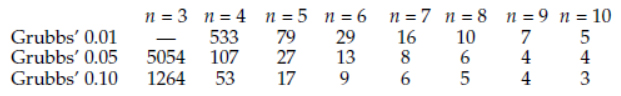Figure 8: Number of measurement increments in std. dev. needed to Use Grubbs’ test

Thus, in general, in order to use Grubbs’ test for n = 5, 6, or 7, you will need measurement increments that are at least one to two orders of magnitude smaller than the standard deviation statistic.

For example, if a set of n = 5 data had a standard deviation statistic of 4.56 units, and if the data were all recorded to the nearest 0.05 units, then the measurement increment would be 0.05 units and the standard deviation would be:and we could use Grubbs’ test at the 0.01 level with these data.

### Dixon’s test for gaps

Two other tests that also strike a balance between finding outliers and preserving good data are Dixon’s test and the W-ratio test. These tests were discussed in earlier articles4,5,6. They differ from the tests above in that they are designed to find a gap in ordered data sets rather than looking for any and all outliers. Figure 9 shows the PPV curves for Dixon’s test. Since the W-ratio test has power curves that are very similar to those of Dixon's test we expect the PPV curves for the W-ratio to be very close to those shown in figure 9.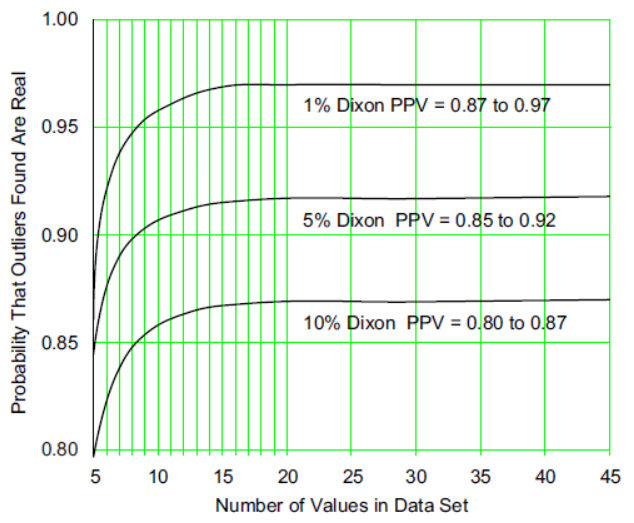Figure 9: PPV curves for Dixon’s test for outliers

Just as the measurement increment can create round-off issues with Grubbs’ test, the same thing happens with Dixon’s test and the W-ratio test. Both of these tests use the global range statistic for the set of n data.

Global Range = Maximum of the n data – Minimum of the n data

In order for Dixon’s test and the W-ratio test to have an overall alpha level that is close to the specified alpha level the global range will have to be greater than the number of measurement increments specified in figure 104.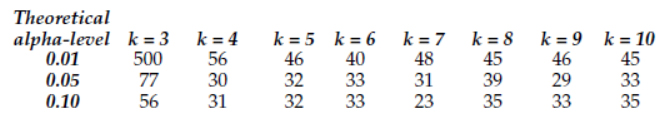Figure 10: Minimum number of measurement increments in global range for robustness

To use Dixon’s test or the W-ratio test at the alpha = 0.01 level you need a range statistic that exceeds roughly 50 measurement increments for n ≥ 4. For larger alpha levels you need a range statistic that exceeds roughly 30 measurement increments for n ≥ 4.

### Comparing the outlier tests

Figure 11 shows the PPV curves for all of the tests considered in both parts of this survey.Figure 11:
PPV curves

The convergence between the curves for the ANOX and Grubbs’ tests tells us that they are going to perform an equivalent job in practice. Dixon’s curves (and those for the W-ratio which are not shown) are slightly below the other curves because they look for gaps instead of extreme values. However, the similarity of all these curves implies that these fixed overall alpha level tests all operate close to the absolute limit of what can be extracted from the data.

This means that other tests for detecting outliers simply cannot do any better job than these three approaches. While other tests may be dressed in different formulas, they will ultimately be either equivalent to ANOX, Grubbs, and Dixon, or they will be inferior to ANOX, Grubbs, and Dixon.

For example the modified Thompson’s tau test is simply Grubbs’ test performed with an overall alpha level that is n times larger than Grubbs’ overall alpha level. As may be seen in figure 11, the effect of increasing the overall alpha level is to lower the PPV curve. Thus, the modified Thompson’s tau test is going to always be inferior to Grubbs’ test. It may find more potential outliers, but it will also have an excessive number of false alarms, undermining your faith in the reality of the potential outliers while removing good data. Such is the quid pro quo required of all such tests.

### Summary

Trying to identify all of the outliers is an unrealistic goal. Likewise, trying to avoid all false alarms is also an unrealistic goal. The trick is to strike a balance between these two goals: Identify those outliers that have a large effect while avoiding false alarms as much as possible.

Procedures that try to capture all of the outliers will go overboard and include good data in the dragnet along with the outliers. As shown in part one this is what happens with Peirce’s test, Chauvenet’s test, and the IQR test.

Procedures that keep the overall alpha level reasonably small will still find the major outliers without an undue increase in risk of false alarms. As shown, the XmR test, ANOX, Grubbs’ test, and the Dixon and W-ratio tests all fall into this category.

Statistical inference is built on the assumption of homogeneous data. Outliers create a lack of homogeneity. In the rush to use their computerized computations people are going to continue to be interested in deleting the outliers.

The problem with deleting the outliers to obtain a homogeneous data set is that the resulting data set will no longer belong to this world. If the analysis of a purified data set ignores the assignable causes that lurk behind most outliers the results will not apply to the underlying process that produces the data. The real question about outliers is not how to get them out of the data, but why do they exist in the first place.

In this author’s 50 years of experience in helping people analyze data, the more profound question has always been “Why are there outliers?” rather than “What do we find when we delete the outliers?”

There are many more tests for outliers, some with sopisticated mathematical theory behind them. Undoubtedly more tests will be created in the future. Many of these will follow Peirce and Chauvenet down the rabbit hole of trying to find all of the outliers so as to obtain a purified data set for their analysis. However, information theory places an upper bound on how much can be extracted from a given data set, and adding more tests will not change this upper bound. ANOX, Grubbs, Dixon, and the W-ratio all approach this upper bound. Other tests can do no better.

So, rather than arguing over which outlier test to use, it is better to find fewer outliers and to discover what happened to create those outliers than it is to find more outliers and delete them in order to analyze data that no longer describe reality.

References

1. Donald J. Wheeler and James Beagle III, “ANOX: The Analysis of Individual Values,”
Quality Digest, September 4, 2017.

2. Frank E. Grubbs, “Sample Criteria for Testing Outlying Observations,”
Annals of Mathematical Statistics, v.21(1), pp. 27-58, 1950.

3. R.E. Shiffler, “Maximum z-Scores and Outliers,”
American Statistician, v. 42, pp. 79-80, 1988.

4. Donald J. Wheeler, “A Problem with Outlier Tests,”
Quality Digest Daily, September 1, 2014.

5. Donald J. Wheeler, “Analysis Using Few Data,” Quality Digest Daily, June 6, 2012.

6. Donald J. Wheeler, “Analysis Using Few Data: Part Two”
Quality Digest Daily, Nov. 5, 2012.

### Discuss### Donald J. Wheeler

Dr. Wheeler is a fellow of both the American Statistical Association and the American Society for Quality who has taught more than 1,000 seminars in 17 countries on six continents. He welcomes your questions; you can contact him at djwheeler@spcpress.com.